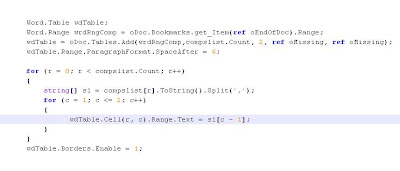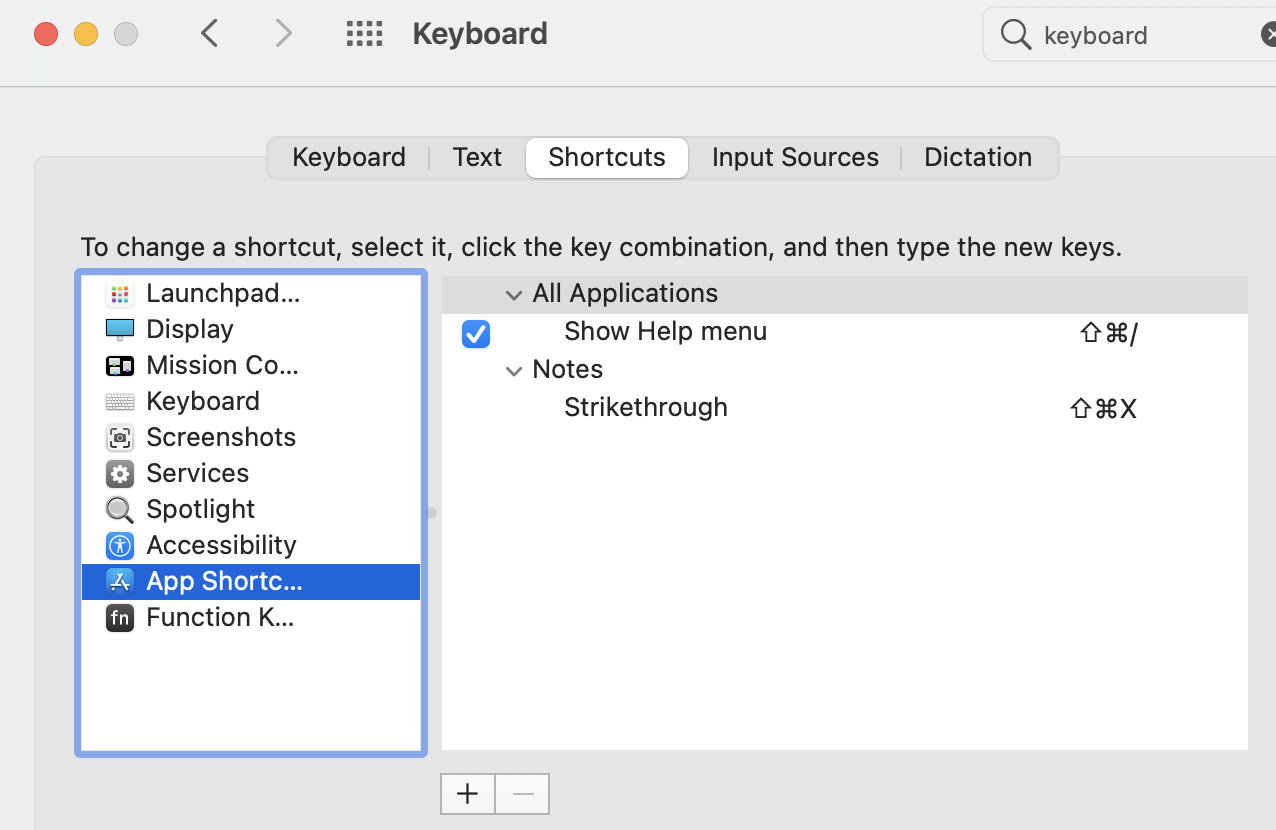### Listing details of files and folders in a Directory in c#

You can get the list of files and folders in WIndows explorer or in a DOS prompt. It's often useful to get content of a folder dynamically using some scripts or programs, especially if you need these details in some applications. Below is a chunk of C# code which gives you the details for files and folders of a given path. You can add additional fields to your out based on your need.

Make an executable file, i.e exe, and use it in your application or run it from the command prompt. Results can be redirected to a text file or csv file.

using System;
using System.Collections.Generic;
using System.Linq;
using System.Text;
using System.IO;

namespace WinConsole
{
class ListFilesOfDirectory
{
public static long size = 0;
public static long totalSizeInMB = 0;
public static long maxSizeInMB = 0;

public static void listFiles(string path)
{

DirectoryInfo di = new DirectoryInfo(path);

DirectoryInfo[] folders = di.GetDirectories();

long[] listOfDir = new long[folders.Length];
int i=0;
foreach (DirectoryInfo d in folders)
{
size = 0;
listOfDir[i] = GetSizeOdDirectory(d);
i += 1;
totalSizeInMB += size / (1024 * 1024);
if (maxSizeInMB <>
maxSizeInMB = size / (1024 * 1024);
}
// display results in tab separated txt file
Console.WriteLine("**\t**\t**\t**\t**");
Console.WriteLine("File\t\t\t\t Size in MB");
for(int j=0;j
{
Console.WriteLine(folders[j].Name + "\t \t \t"+listOfDir[j].ToString());
}
Console.WriteLine("**\t**\t**\t**\t**");
}

public static long GetSizeOdDirectory(DirectoryInfo directory)
{

DirectoryInfo[] dir = directory.GetDirectories();
foreach (DirectoryInfo d in dir)
{
GetSizeOdDirectory(d);
}
FileInfo[] files = directory.GetFiles();
foreach (FileInfo f in files)
{
size += f.Length;
}
return size;
}
public static void Main(string[] args)
{
string path=args.ToString();
listFiles(path);

}
}
}

### The server threw an exception. (Exception from HRESULT: 0x80010105 (RPC_E_SERVERFAULT)) MS Word automation solution

I was frustrated by this error every time I run the below code. I did a lot of googling nothing worked. Even Microsoft said it a known issue we don't recommend this.
Then later just to try I just put one try-catch block and it worked perfectly. To date I have not seen that error.### Convert currency to word ( text ) in excel 2.0

Update: New version 3.0 has been released with bug fixes.

Version 3.0

Thank you guys for reported bugs in the code (Convert currency to word in excel 1.0) in my earlier post Convert currency to word in excel.

Your comments helped me a lot in fixing this error. There is a new version 2.0 which not only has bug fixes but also can convert up to 1,234,567,890.00.

Keep commenting

### Convert currency to word in excel

There are plenty of online resources to convert Numeric currencies to Text- from \$200 to Dollar Two Hundred.
But this is applicable only ###,###,### (comma at every third number)format.  This is not suitable for India numbering system, because we follow ##,##,###(....22,22,222) format.

So I have create a excel function to over come this. You can use this function to to convert number up to 10,00,00,000(ten crores).

How to do it

Open Excel

Press Alt + F11

Copy paste the This Module there.

Exit from VBA window by pressing Alt + F11 again

In excel sheet, Insert Function,

Under user defined find ConvertCurrencyToEnglish

select it and provide the number as a parameter

thats all you are done
----------------------------------------------------------------------
----------------------------------------------------------------------

' ****  Author                 : Krishna S
' ****  Tittle                    : Converting Hindu Arabic Currency(Indian System) to Words
' ****  Description          : This utility converts currencies in Indian numbering system to words.
' ****  Limitations           : Converts only upto 10,00,00,000( Ten Crores)

Function ConvertCurrencyToEnglish(ByVal MyNumber)
Dim Temp
Dim Rupees, Paise
Dim DecimalPlace, Count
ReDim Place(9) As String
Place(2) = " Thousand "
Place(3) = " Lac "
Place(4) = " Core "
'   Place(5) = " Hundred Core "
' Convert MyNumber to a string, trimming extra spaces.
MyNumber = Trim(Str(MyNumber))
' Find decimal place.
DecimalPlace = InStr(MyNumber, ".")
' If we find decimal place...
If DecimalPlace > 0 Then
' Convert Paise
Temp = Left(Mid(MyNumber, DecimalPlace + 1) & "00", 2)
Paise = ConvertTens(Temp)
' Strip off Paise from remainder to convert.
MyNumber = Trim(Left(MyNumber, DecimalPlace - 1))
End If
Count = 1

Do While MyNumber <> ""
If Count = 1 Then

Temp = ConvertHundreds(Right(MyNumber, 3))

If Temp <> "" Then Rupees = Temp & Place(Count) & Rupees
If Len(MyNumber) > 3 Then
' Remove last 3 converted digits from MyNumber.
MyNumber = Left(MyNumber, Len(MyNumber) - 3)
Else
MyNumber = ""
End If
Count = Count + 1
Else
' Convert last 3 digits of MyNumber to English Rupees.
Temp = ConvertTens(Right(MyNumber, 2))

If Temp <> "" Then Rupees = Temp & Place(Count) & Rupees
If Len(MyNumber) > 3 Then
' Remove last 3 converted digits from MyNumber.
MyNumber = Left(MyNumber, Len(MyNumber) - 2)
Else
MyNumber = ""
End If
Count = Count + 1
End If
Loop
' Clean up Rupees.
Select Case Rupees
Case ""
Rupees = ""
Case "One"
Rupees = "One Rupee"
Case Else
Rupees = Rupees & " Rupees"
End Select
' Clean up Paise.
Select Case Paise
Case ""
Paise = ""
Case "One"
Paise = " And One Cent"
Case Else
Paise = " And " & Paise & " Paise"
End Select
ConvertCurrencyToEnglish = Rupees & Paise
End Function
Private Function ConvertHundreds(ByVal MyNumber)
Dim Result As String
' Exit if there is nothing to convert.
If Val(MyNumber) = 0 Then Exit Function
' Append leading zeros to number.
MyNumber = Right("000" & MyNumber, 3)
' Do we have a hundreds place digit to convert?
If Left(MyNumber, 1) <> "0" Then
Result = ConvertDigit(Left(MyNumber, 1)) & " Hundred "
End If
' Do we have a tens place digit to convert?
If Mid(MyNumber, 2, 1) <> "0" Then
Result = Result & ConvertTens(Mid(MyNumber, 2))
Else
' If not, then convert the ones place digit.
Result = Result & ConvertDigit(Mid(MyNumber, 3))
End If
ConvertHundreds = Trim(Result)
End Function
Private Function ConvertTens(ByVal MyTens)
Dim Result As String
' Is value between 10 and 19?
If Val(Left(MyTens, 1)) = 1 Then
Select Case Val(MyTens)
Case 10: Result = "Ten"
Case 11: Result = "Eleven"
Case 12: Result = "Twelve"
Case 13: Result = "Thirteen"
Case 14: Result = "Fourteen"
Case 15: Result = "Fifteen"
Case 16: Result = "Sixteen"
Case 17: Result = "Seventeen"
Case 18: Result = "Eighteen"
Case 19: Result = "Nineteen"
Case Else
End Select
Else
' .. otherwise it's between 20 and 99.
Select Case Val(Left(MyTens, 1))
Case 2: Result = "Twenty "
Case 3: Result = "Thirty "
Case 4: Result = "Forty "
Case 5: Result = "Fifty "
Case 6: Result = "Sixty "
Case 7: Result = "Seventy "
Case 8: Result = "Eighty "
Case 9: Result = "Ninety "
Case Else
End Select
' Convert ones place digit.
Result = Result & ConvertDigit(Right(MyTens, 1))
End If
ConvertTens = Result
End Function
Private Function ConvertDigit(ByVal MyDigit)
Select Case Val(MyDigit)
Case 1: ConvertDigit = "One"
Case 2: ConvertDigit = "Two"
Case 3: ConvertDigit = "Three"
Case 4: ConvertDigit = "Four"
Case 5: ConvertDigit = "Five"
Case 6: ConvertDigit = "Six"
Case 7: ConvertDigit = "Seven"
Case 8: ConvertDigit = "Eight"
Case 9: ConvertDigit = "Nine"
Case Else: ConvertDigit = ""
End Select
End Function
-----------------------------------------------------------

### Apple MacBook Notes strike through Menu option and Setting Keyboard shortcut.

Apple Notes is the probably most widely used App for taking notes on Apple MacBook or Apple Mac in general. In this tutorial, I will show y...